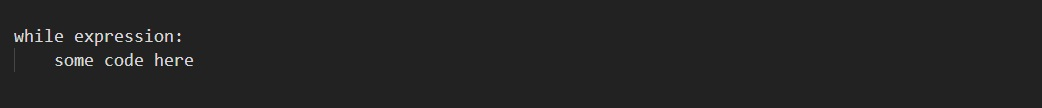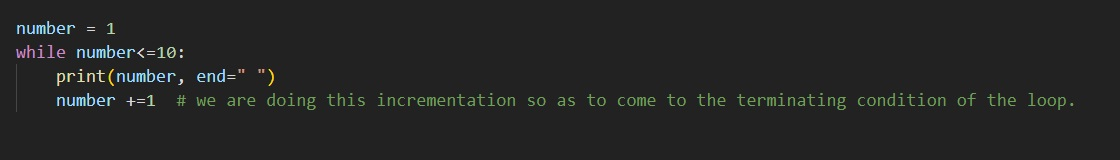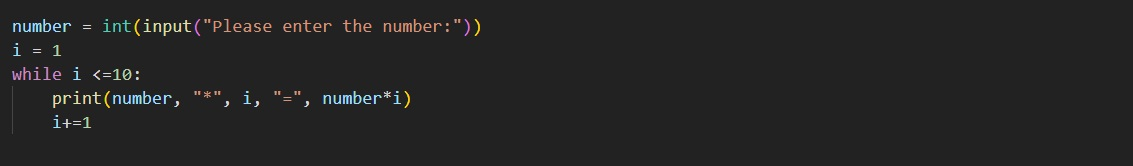# Python while loop

Python while loop

Now, exploring the concept of looping, Let’s have a look at the while loop now. Basically, the loops are used when we need to execute something repeatedly, and the while loop executes some block of code, as long as the given expression evaluates to True. This is like – while this is True, do that (that is basically some block of code, which we want to execute).

Now, first of all, Let’s have a look at the syntax of the while loop, so that we can simply move forward, understanding the working of the while loop.As you can see, we have the while keyword, and after that, we have some expression. Now, the while loop is going to execute as long as the expression evaluates to True. At times, there are situations, when we need to use the while loop. Now, Let’s have a look at a very simple program, where we will simply print numbers from 1 to 10, using the while loop. Let’s have a look at the program –As you can see in the above program, we have a variable number, with value assigned as 1, and then we have the while loop, with the expression, which says number less than or equal to 10. this simply means now, that we are going to do something, while the number value is less than or equal to 10. this is very simple and readable. Then, we are printing the number, and incrementing it, so that we gradually come closer to the terminating condition of the loop, so that the condition somewhere becomes False, and then we are out of the loop. The output of the above program comes out to be something like this –

1 2 3 4 5 6 7 8 9 10

As you can see, we got the numbers from 1 to 10. Now, Let’s understand how the while loop works. Basically, the idea is that the while loop executes as long as the expression evaluates to True. So, every time, the expression is checked first, and if it evaluates to True, then only we are going to enter the body of the loop. Again in the next iteration, the expression is checked first, and once the expression evaluates to False, we are out of the loop.

Remember that here as well, the body is determined using the indentations. The indentations play a very important role here, so you just have to take care of the indents that you are giving.

Now, Let’s have a look at a simple example, with which, we will become more familiar with the concept of the while loop. In the below program, Let’s print the table of some given number. We will get the number as a user input.As you can see in the above program, we are getting the number as a user input. With the while loop, we have the expression, which says that i<=10, and we have the variable i initialized to 1. From this, it is clear that we are going to do something, while the value of the i variable is less than or equal to 10. Also, in the body of the while loop, we are printing the output, and then we are incrementing the value of the variable i, so that gradually we come to the terminating condition. The output of the above program comes out to be something like this –

14 * 1 = 14
14 * 2 = 28
14 * 3 = 42
14 * 4 = 56
14 * 5 = 70
14 * 6 = 84
14 * 7 = 98
14 * 8 = 112
14 * 9 = 126
14 * 10 = 140

As you can see in the above program, we are simply getting the table of some number as an output.

You can try this program for some other values as well. Also, the thing is that we are incrementing the value of i in the loop, and we are doing this so that we reach to the terminating condition. But what if we forgot to do that? Well, the simple answer to this question is that the loop will never end.

The thing is that the while loop executes as long as the expression evaluates to True. But the thing is that if the condition was never False, then the loop will run for infinite. So, it is important to look after the terminating conditions, and how we can get to that.

So, we can simply make use of the while loop, as and when required in our python programs. You can practice some more programs related to the while loop, and the looping concept overall, so as to get familiar with the concept, and be comfortable using the loops in the program.